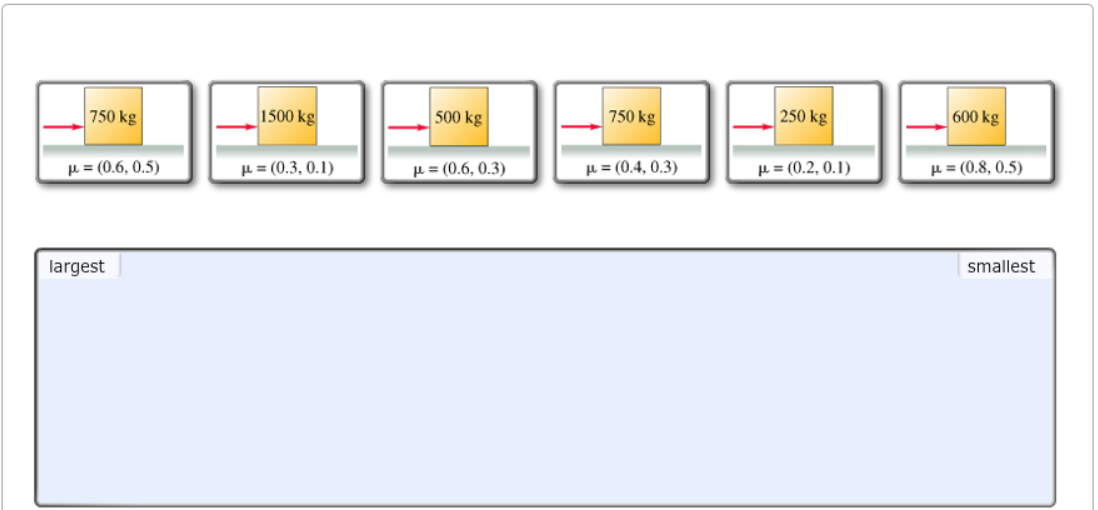# Problem: Rank the crates on the basis of the kinetic frictional force acting on them. Rank from largest to smallest. To rank items as equivalent, overlap them.

###### FREE Expert Solution

Friction force:

$\overline{)\begin{array}{rcl}{\mathbf{f}}& {\mathbf{=}}& {\mathbf{\mu N}}\\ {\mathbf{f}}& {\mathbf{=}}& {\mathbf{\mu mg}}\end{array}}$

Ranking based on kinetic friction:

For 1, fk = (0.5)(750)(9.81) = 3679 N###### Problem Details

Rank the crates on the basis of the kinetic frictional force acting on them. Rank from largest to smallest. To rank items as equivalent, overlap them.